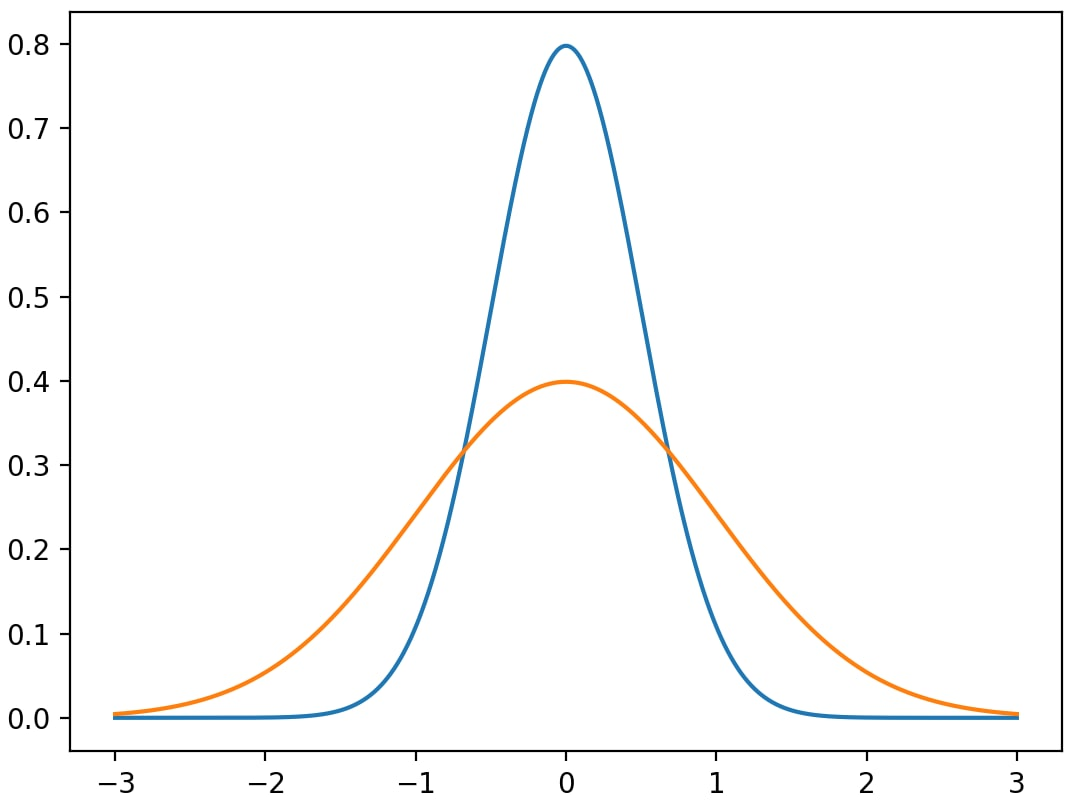# Example Diagnostic Documentation¶

Last update: 5/06/2020

This is an example document that you can use as a template for your diagnostics’ own documentation: it describes what information you should provide in each section. For example, if this were a real POD, you’d place a one-paragraph synopsis of your diagnostic here (like an abstract).

It also serves as an example of the RestructuredText (ReST, .rst) format used to generate this page: compare this output with the input source file. The easiest way to get started is to copy the source file into the online editor at https://livesphinx.herokuapp.com/ and experiment.

## Version & Contact info¶

Here you should describe who contributed to the diagnostic, and who should be contacted for further information:

• Version/revision information: version 1 (5/06/2020)

• PI (name, affiliation, email)

• Developer/point of contact (name, affiliation, email)

• Other contributors

## Functionality¶

In this section you should summarize the stages of the calculations your diagnostic performs, and how they translate to the individual source code files provided in your submission. This will, e.g., let maintainers fixing a bug or people with questions about how your code works know where to look.

## Required programming language and libraries¶

In this section you should summarize the programming languages and third-party libraries used by your diagnostic. You also provide this information in the settings.jsonc file, but here you can give helpful comments to human maintainers (eg, “We need at least version 1.5 of this library because we call this function.”)

## Required model output variables¶

In this section you should describe each variable in the input data your diagnostic uses. You also need to provide this in the settings.jsonc file, but here you should go into detail on the assumptions your diagnostic makes about the structure of the data.

In this section, you can go into more detail on the science behind your diagnostic, for example, by copying in relevant text articles you’ve written using th It’s especially helpful if you’re able to teach users how to use your diagnostic’s output, by showing how to interpret example plots.

Instead of doing that here, we provide more examples of RestructuredText syntax that you can customize as needed.

As mentioned above, we recommend the online editor at https://livesphinx.herokuapp.com/, which gives immediate feedback and has support for sphinx-specific commands.

Here’s an introduction to the RestructuredText format, a quick reference, and a syntax comparison to other text formats you may be familiar with.

### More references and citations¶

Here’s another reference:

.. _ref-Charney:

2. Charney, Jule; Fjørtoft, Ragnar; von Neumann, John (1950). Numerical
Integration of the Barotropic Vorticity Equation. *Tellus* **2** (4) 237–254,
doi:10.3402/tellusa.v2i4.8607 <https://doi.org/10.3402/tellusa.v2i4.8607>__.


2. Charney, Jule; Fjørtoft, Ragnar; von Neumann, John (1950). Numerical Integration of the Barotropic Vorticity Equation. Tellus 2 (4) 237–254, doi:10.3402/tellusa.v2i4.8607.

Here’s an example of citing these references:

:ref:Maloney et. al., 2019 <ref-Maloney>,
:ref:Charney, Fjørtoft and von Neumann, 1950 <ref-Charney>


### Figures¶

Images must be provided in either .png or .jpeg formats in order to be displayed properly in both the html and pdf output.

Here’s the syntax for including a figure in the document:

.. _my-figure-tag: [only needed for linking to figures]

.. figure:: [path to image file, relative to the source.rst file]
:align: left
:width: 75 % [these both need to be indented by three spaces]

Paragraphs or other text following the figure that are indented by three
spaces are treated as a caption/legend, eg:

- red line: a Gaussian
- blue line: another Gaussian


which producesParagraphs or other text following the figure that are indented by three spaces are treated as a caption/legend, eg:¶ blue line: a Gaussian orange line: another Gaussian

The tag lets you refer to figures in the text, e.g. :ref:Figure 1 <my-figure-tag>Figure 1.

### Equations¶

Accented and Greek letters can be written directly using Unicode: é, Ω. (Make sure your text editor is saving the file in UTF-8 encoding).

Use the following syntax for superscripts and subscripts in in-line text:

W m\ :sup:-2\ ; CO\ :sub:2\ .


which produces: W m-2; CO2. Note one space is needed after both forward slashes in the input; these spaces are not included in the output.

Equations can be written using standard latex (PDF link) syntax. Short equations in-line with the text can be written as :math:f = 2 \Omega \sin \phi$$f = 2 \Omega \sin \phi$$.

Longer display equations can be written as follows. Note that a blank line is needed after the .. math:: heading and after each equation, with the exception of aligned equations.

.. math::

\frac{D \mathbf{u}_g}{Dt} + f_0 \hat{\mathbf{k}} \times \mathbf{u}_a &= 0; \\
\frac{Dh}{Dt} + f \nabla_z \cdot \mathbf{u}_a &= 0,

\text{where } \mathbf{u}_g = \frac{g}{f_0} \hat{\mathbf{k}} \times \nabla_z h.


which produces:

\begin{align}\begin{aligned}\begin{split}\frac{D \mathbf{u}_g}{Dt} + f_0 \hat{\mathbf{k}} \times \mathbf{u}_a &= 0; \\ \frac{Dh}{Dt} + f \nabla_z \cdot \mathbf{u}_a &= 0,\end{split}\\\text{where } \mathbf{u}_g = \frac{g}{f_0} \hat{\mathbf{k}} \times \nabla_z h.\end{aligned}\end{align}

The editor at https://livesphinx.herokuapp.com/ can have issues formatting complicated equations, so you may want to check its output with a latex-specific editor, such as overleaf or other equation editors.